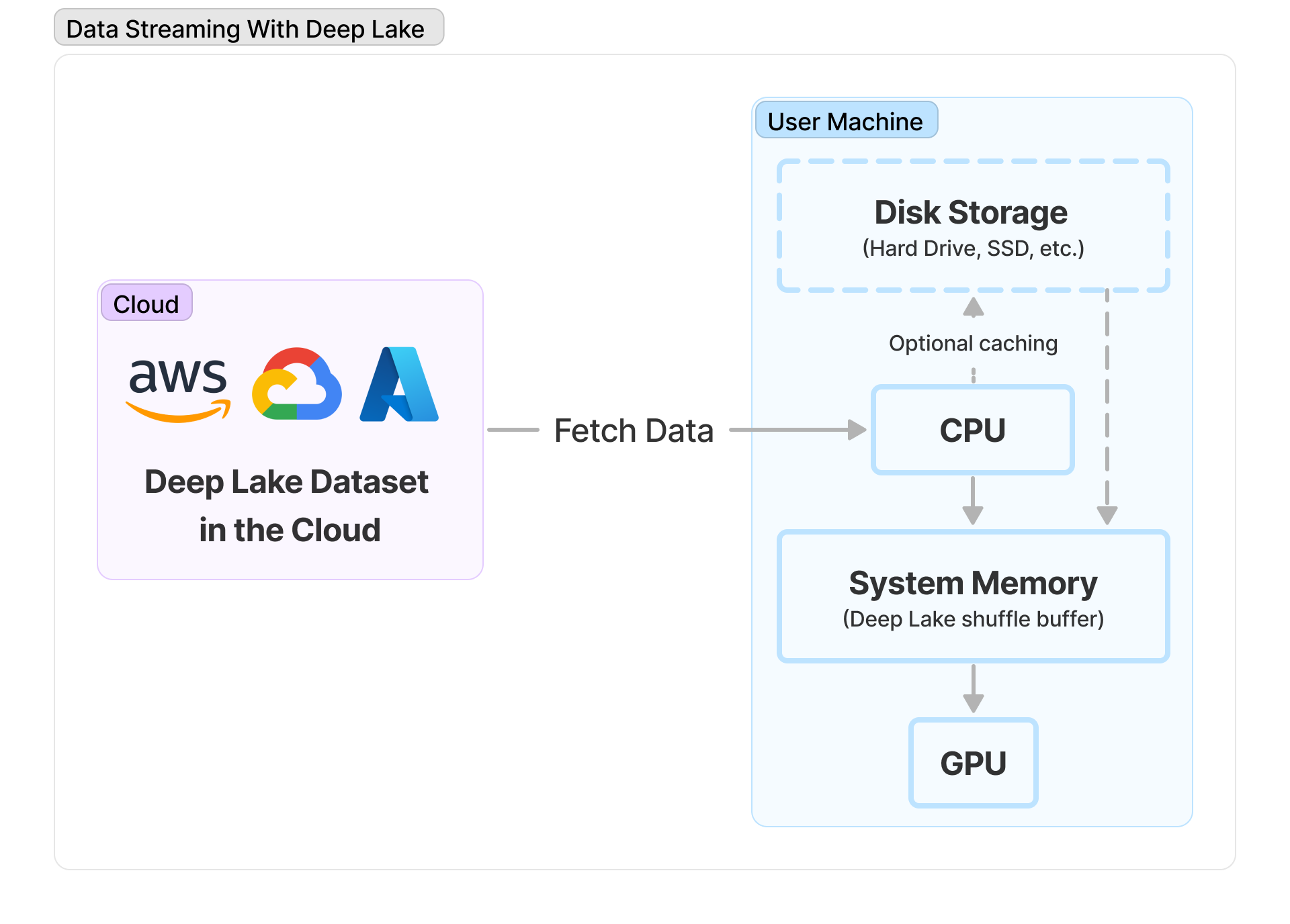Comment on page

# Step 7: Connecting Deep Lake Datasets to ML Frameworks

Connecting Deep Lake Datasets to machine learning frameworks such as PyTorch and TensorFlow.

## How to use Deeplake with PyTorch or TensorFlow in Python

Deep Lake Datasets can be connected to popular ML frameworks such as PyTorch and TensorFlow, so you can train models while streaming data from the cloud without bottlenecking the training process!Data Streaming using Deep Lake

### Training models with PyTorch

There are two syntaxes that can be used to train models in Pytorch using Deep Lake datasets:
1. 1.
Deep Lake Data Loaders are highly-optimized and unlock the fastest streaming and shuffling using Deep Lake's internal shuffling method. However, they do not support custom sampling or fully-random shuffling that is possible using PyTorch datasets + data loaders.
2. 2.
Pytorch Datasets + PyTorch Data Loaders enable all the customizability supported by PyTorch. However, they have highly sub-optimal streaming using Deep Lake datasets and may result in 5X+ slower performance compared to using Deep Lake data loaders.

### 1. Deep Lake Data Loaders for PyTorch

Best option for fast streaming!
The fastest streaming of data to GPUs using PyTorch is achieved using Deep Lake's built-in PyTorch dataloader `ds.pytorch()` . If your model training is highly sensitive to the randomization of the input data, please pre-shuffle the data, or explore our writeup on.
import deeplake
from torchvision import datasets, transforms, models
ds = deeplake.load('hub://activeloop/cifar100-train') # Deep Lake Dataset

#### Transform syntax #1 - For independent transforms per tensor

The `transform` parameter in `ds.pytorch()` is a dictionary where the `key` is the tensor name and the `value` is the transformation function for that tensor. If a tensor does not need to be returned, the tensor should be omitted from the keys. If no transformation is necessary on a tensor, the transformation function is set as `None`.
tform = transforms.Compose([
transforms.ToPILImage(), # Must convert to PIL image for subsequent operations to run
transforms.RandomRotation(20), # Image augmentation
transforms.ToTensor(), # Must convert to pytorch tensor for subsequent operations to run
transforms.Normalize([0.5, 0.5, 0.5], [0.5, 0.5, 0.5]),
])
dataloader= ds.pytorch(batch_size = 16, num_workers = 2,
transform = {'images': tform, 'labels': None}, shuffle = True)

#### Transform syntax #2 - For complex or dependent transforms per tensor

Sometimes a single transformation function might need to be applied to all tensors, or tensors need to be combined in a transform. In this case, you can use the syntax below to perform the exact same transform as above:
def transform(sample_in):
return {'images': tform(sample_in['images']), 'labels': sample_in['labels']}
dataloader= ds.pytorch(batch_size = 16, num_workers = 2,
transform = transform,
tensors = ['images', 'labels'],
shuffle = True)
Some datasets such as ImageNet contain both grayscale and color images, which can cause errors when the transformed images are passed to the model. To convert only the grayscale images to color format, you can add this Torchvision transform to your pipeline:
`transforms.Lambda(lambda x: x.repeat(int(3/x.shape), 1, 1))`

### 2. PyTorch Datasets + PyTorch Data Loaders using Deep Lake

Best option for full customizability.
Deep Lake datasets can be integrated in the PyTorch Dataset class by passing the `ds` object to the PyTorch Dataset's constructor and pulling data in the `__getitem__` method using `self.ds.image[ids].numpy()`:
class ClassificationDataset(Dataset):
def __init__(self, ds, transform = None):
self.ds = ds
self.transform = transform
def __len__(self):
return len(self.ds)
def __getitem__(self, idx):
image = self.ds.images[idx].numpy()
label = self.ds.labels[idx].numpy(fetch_chunks = True).astype(np.int32)
if self.transform is not None:
image = self.transform(image)
sample = {"images": image, "labels": label}
return sample
When loading data sequentially, or when randomly loading samples from a tensor that fits into the cache (such as `class_labels`) it is recommended to set `fetch_chunks = True`. This increases the data loading speed by avoiding separate requests for each individual sample. This is not recommended when randomly loading large tensors, because the data is deleted from the cache before adjacent samples from a chunk are used.
The PyTorch dataset + data loader is instantiated using the built-in PyTorch functions:
cifar100_pytorch = ClassificationDataset(ds_train, transform = tform)
dataloader_pytroch = DataLoader(dataset_pt, batch_size = 16, num_workers = 2, shuffle = True)

### Iteration and Training

You can iterate through both data loaders above using the exact same syntax. Loading the first batch of data using the Deep Lake data loader may take up to 30 seconds because the shuffle buffer is filled before any data is returned.
print(data)
break
# Training Loop
Deep Lake Datasets can be converted to TensorFlow Datasets using `ds.tensorflow()`. Downstream, functions from the `tf.Data` API such as map, shuffle, etc. can be applied to process the data before training.